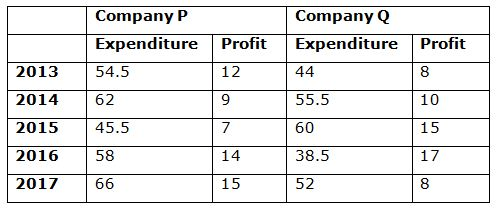# IBPS SO / NIACL AO Prelims – Quantitative Aptitude Questions Day- 64

Dear Readers, Bank Exam Race for the Year 2018 is already started, To enrich your preparation here we have providing new series of Practice Questions on Quantitative Aptitude – Section. Candidates those who are preparing for IBPS SO / NIACL AO Prelims 2018 Exams can practice these questions daily and make your preparation effective.

[WpProQuiz 4725]

Directions (Q. 1 – 5): In the following questions, two equations I and II are given. You have to solve both the equations and give Answer as,

a) If x > y

b) If x ≥ y

c) If x < y

d) If x ≤ y

e) If x = y or the relation cannot be established

1)

I) 5x2 – 22x -15 = 0

II) 4y2 – 24y – 13 = 0

2)

I) 2x2 + 19x + 45 = 0

II) y2 – 17y + 72 = 0

3)

I) 3/√x + 7/√x = √x

II) y2 = 125/2/ √y

4)

I) 3x = 5y – 4

II) 5x + 7y – 70 = 0

5)

I) x2 = 14161

II) y = √14161

Directions (Q. 6 – 10): Study the following information carefully and answer the given questions:

The following table shows the expenditure and profit (In lakhs) of two different companies P and Q in five different years.Note: Income = Expenditure + Profit

6) Total income of Company P in the year 2013 and 2017 together is approximately what percentage of total income of Company Q in the year 2014 and 2016 together?

a) 145 %

b) 134 %

c) 122 %

d) 113 %

e) 98 %

7) Find the ratio between the profits of Company P to that of Company Q in all the given years together?

a) 34: 39

b) 57: 58

c) 15: 17

d) 23: 25

e) None of these

8) Find the average of Expenditure of company Q in the year 2013, 2014 and 2016 together?

a) 38 lakhs

b) 44 lakhs

c) 42 lakhs

d) 46 lakhs

e) None of these

9) Find the difference between the expenditure of company P in the year 2013, 2015 and 2017 together to that of the expenditure of Company Q in the year 2014, 2015 and 2016 together?

a) 12 lakhs

b) 18 lakhs

c) 24 lakhs

d) 26 lakhs

e) None of these

10) Total income of Company Q in the year 2017 is what percentage of total income of same company in the year 2015?

a) 95 %

b) 75 %

c) 110 %

d) 80 %

e) None of these

I) 5x2 – 22x -15 = 0

5x2 – 25x + 3x -15 = 0

5x(x – 5) + 3(x – 5) = 0

(5x + 3) (x – 5) = 0

X = -3/5, 5 = -0.6, 5

II) 4y2 – 24y – 13 = 0

4y2 + 2y – 26y – 13 = 0

2y (2y + 1) – 13(2y + 1) = 0

(2y – 13) (2y + 1) = 0

Y = 13/2, -1/2 = 6.5, -0.5

Can’t be determined

I) 2x2 + 19x + 45 = 0

2x2 + 10x+ 9x + 45 = 0

2x(x + 5) + 9 (x + 5) = 0

(2x + 9) (x + 5) = 0

X = -9/2, -5 = -4.5, -5

II) y2 – 17y + 72 = 0

y2 – 8y – 9y + 72 = 0

y(y – 8) – 9(y – 9) = 0

(y – 8) (y – 9) = 0

Y = 8, 9

X < y

I) 3/√x + 7/√x = √x

(3 + 7)/ √x = √x

X = 10

II) y2 = 125/2/ √y

y2 × √y = 125/2

y2 + (1/2) = 125/2

y5/2 = 125/2

y = 12

X < y

3x – 5y = 4–> (1)

5x + 7y = 70–>(2)

By solving the equation (1) and (2),

X = 7, y = 5

X > y

I) x2 = 14161

X = +119, -119

II) y = √14161

Y = 119

X ≤ y

Directions (6-10):

Total income of Company P in the year 2013 and 2017 together

= > (54.5 + 12) + (66 + 15) = 66.5 + 81

= > 147.5 lakhs

Total income of Company Q in the year 2014 and 2016 together

= > (55.5 + 10) + (38.5 + 17) = 65.5 + 55.5

= > 121 lakhs

Required % = (147.5/121)*100 = 122 %

The profit of Company P in all the given years together

= > 12 + 9 + 7 + 14 + 15 = 57 lakhs

The profit of Company Q in all the given years together

= > 8 + 10 + 15 + 17 + 8 = 58 lakhs

Required ratio = 57: 58

The total Expenditure of company Q in the year 2013, 2014 and 2016 together

= > 44 + 55.5 + 38.5 = 138 lakhs

Required average = 138/3 = 46 lakhs

The expenditure of company P in the year 2013, 2015 and 2017 together

= > 54.5 + 45.5 + 66 = 166 lakhs

The expenditure of Company Q in the year 2014, 2015 and 2016 together

= > 55.5 + 60 + 38.5 = 154 lakhs

Required difference = 166 – 154 = 12 lakhs

Total income of Company Q in the year 2017

= > 52 + 8 = 60 lakhs

Total income of Company Q in the year 2015

= > 60 + 15 = 75 lakhs

Required % = (60/75)*100 = 80 %